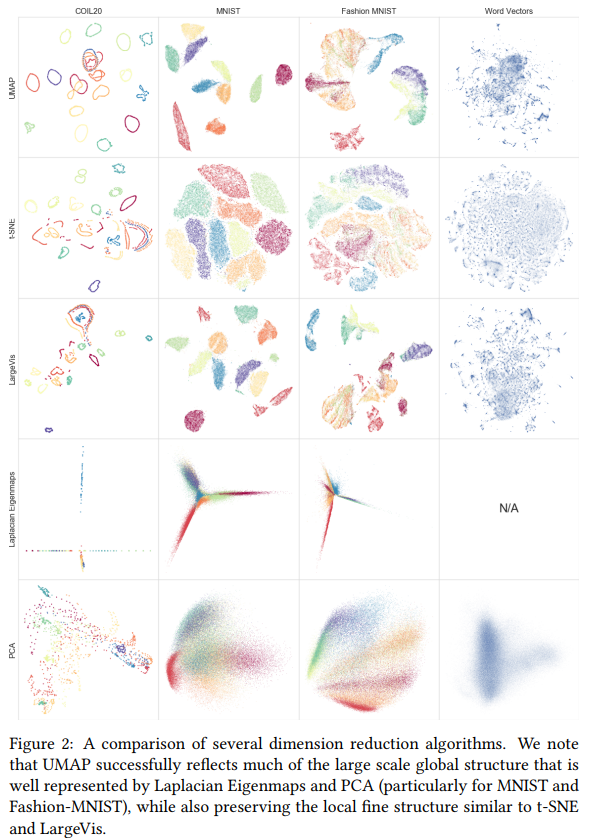Uniform Manifold Approximation and Projection (UMAP) is a new technique for dimensionality reduction in the family of “neighbour graphs” techniques (as opposed to the “matrix factorization” family such as PCA).

The paper is quite math-heavy but here’s the TLDR:

• Let’s assume that our dataset is distributed uniformly on a manifold and that this manifold is locally connected.
• Let’s create a fuzzy metric that forces the “uniform” behaviour on the manifold (a Reimanian metric).
• $$w_i(X_i, X_j) = exp(-(d(X_i, X_j) - \rho_i)/ \sigma_i)$$ where d is a distance function, $$\rho_i$$ is the distance to $$X_i$$ nearest neighbour and $$\sigma_i$$ is the diameter of the neighbourhood.
• Since this metric is not symetrical, the actual metric is : $$f(\alpha, \beta) = \alpha + \beta - \alpha * \beta$$. Where $$alpha = w_i(X_i, X_j)$$ and $$beta = w_j(X_i, X_j)$$.

We then create an embedding $$w'$$ which will embed our data in the lower space (initialized randomly). Finally we optimize the $$w'$$ using the crossentropy between $$w'$$ and $$w$$ using SGD.

$C(w, w') = \sum_{i\sim j} w(i,j)log(\frac{w(i,j)}{w'(i,n)}) + (1 - w(i,j)) log(1 - \frac{w(i,j)}{1 - w'(i,n)})$

Why is this fast?

• Need to compute the k-NN only once.
• The comparison is only done on the neighbours of $$X_i, X_j$$.

Features:

• Fast (it is using Numba for LLVM compilation)
• Can be used on new data points.
• The classes can be provided to get a better embedding while keeping the relation between classes.
• ## Solid theoretical foundation (Category, Topology)

Code

Blog

Awesome Talk